# SSAT Upper Level Math : How to add

## Example Questions

### Example Question #1 : Basic Addition, Subtraction, Multiplication And Division

What is the sum of the two percentages in decimal form?

7 percent and 135 percent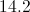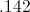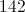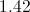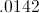Explanation:

You add the percentages and you get 142 percent. To convert a percent to a decimal, you divide by 100, so the answer is 1.42.

### Example Question #1 : Basic Addition, Subtraction, Multiplication And Division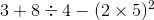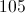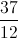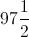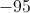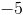Explanation:

Do the expression in the parantheses and you get. Then you do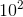and you get. Then you do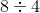and you get. Then you add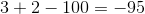.

### Example Question #1 : How To Add And Subtract Decimals

If Susie bought a pair of shoes for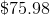, a shirt for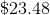, and a pair of pants for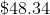. How much did she spend in total?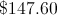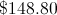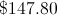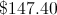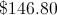Explanation:

You add all three values and you get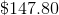.

### Example Question #2 : Basic Addition, Subtraction, Multiplication And Division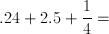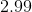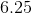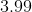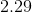Explanation:

First, convert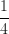into a decimal. We are left with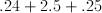Now add the three numbers, being careful to line the decimal points up exactly.  From right to left we will add:

1) 4 and 5 = 9

2) 5, 2, and 2 = 9

3) 2 is alone = 2

This leaves us with 2.99 for the final answer.

### Example Question #1 : Basic Addition, Subtraction, Multiplication And Division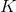andare prime integers: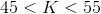and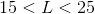. How many possible values of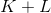are there?

Eight

Four

Seven

Six

Five

Five

Explanation:can be equal to any of the prime numbers between 45 and 55, of which there are two - 47 or 53.can be equal to any of the prime numbers between 15 and 25, of which there are three - 17, 19, and 23.

Their sum can be any of the following: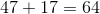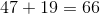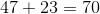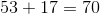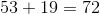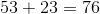This makes five possible sums.

### Example Question #1 : How To Addandare prime integers.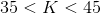and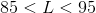. What is the greatest possible value of?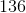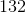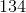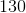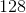Explanation:

To find the greatest possible value of, add the greatest possible value ofto the greatest possible value of. This happens whenis equal to the greatest prime between 35 and 45, which is 43, and whenis equal to the greatest prime between 85 and 95, which is 89. Add the two numbers: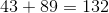### Example Question #1 : Basic Addition, Subtraction, Multiplication And Division

In a math contest, the team from Lincoln High comprised five students - Melanie, Ned, Olivia, Phyllis, and Quinn. Melanie outscored Ned by 12 points; Ned outscored Quinn by 19 points; Phyllis outscored Ned by 45 points and Olivia by 20 points. Who came in third among the five?

Quinn

Olivia

Phyllis

Ned

Melanie

Melanie

Explanation:

Since this reasoning is valid regardless of the actual scores, suppose Quinn scored 100. Then Ned scored 19 more than Quinn, or 119, and Melanie scored 12 more than Ned, or 131. Phyllis scored 45 more than Ned, or 164, and Olivia scored 20 points fewer than Phyllis, or 144. This makes the order, from greatest to least:

Phyllis, Olivia, Melanie, Ned, Quinn

Melanie scored third among the five.

### Example Question #1 : Basic Addition, Subtraction, Multiplication And Division

Which of the following angles is supplementary to an angle that is 45 degrees?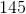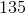Explanation:

Supplementary angles add up to 180 degrees. Given that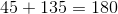, the correct answer is 135.

### Example Question #1 : Basic Addition, Subtraction, Multiplication And Division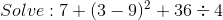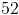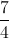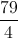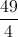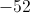Explanation:

Use order of operations, so you would first complete the operation in the parentheses, which would give you.

Then you do exponenets, so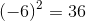.

Then you do division which is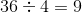.

Then you do addition from left to right, so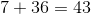.

Last, you add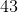to, which is.

### Example Question #2 : Basic Addition, Subtraction, Multiplication And Division

Simplify: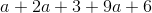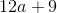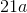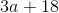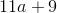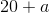Explanation:

To simplify this expression, regroup and combine like-terms.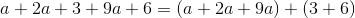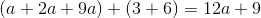### All SSAT Upper Level Math Resources